# Coordinates

3D models are usually made up of thousands or even millions of triangles (or other polygons).

In order to break things down to the simplest case we will enter a single triangle and show what we can do with that.

First insert the following nodes by using the insert button.

insert a Transform Node, under that insert a shape3d node, under that insert an Indexed face Set (IFS) node, under that insert a coordinate node.

In the coordinate node enter some coordinates to represent the triangle as follows.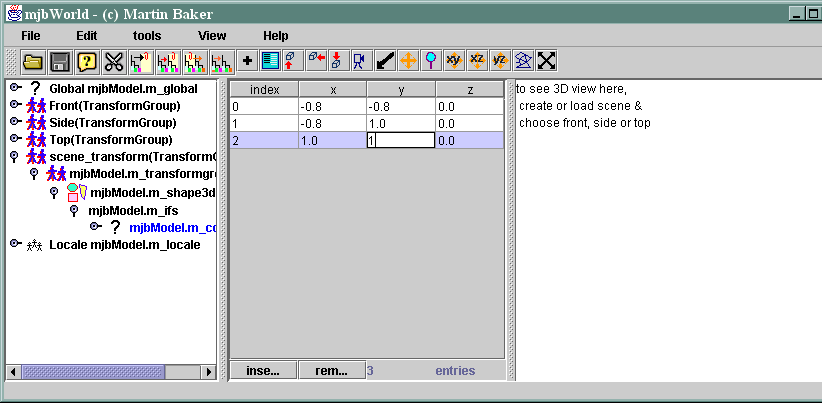Now enter the coordinate indexes. In mjbWorld all geometry values are indexed, this allows each coordinate etc to be patched to a different position, the end of each triangle is marked with -1.

When this is done the 3D window is still black. Why is this?This is because the triangle can only be seen from one side and we are looking from the back. This is determined by the right hand rule. That is if the fingers of the right hand are rotated in the direction of the vertexes, then the thumb will indicate the direction that the face will be seen from.

So we can use the indexes to change the order of the vertexes. They now go round in a clockwise direction and the triangle can be seen.## Colours

Now lets add a colour node under the IFS node. and give it three values as shown below.

(to enter the colour click twice on the colour column which will bring up the colour dialog)

We also need to enter indexes in the IFS for the colourindexes. enter the same values as for the coordinate indexes.

Notice that each vertex is set with the colour that you entered, the values inbetween are interpolated.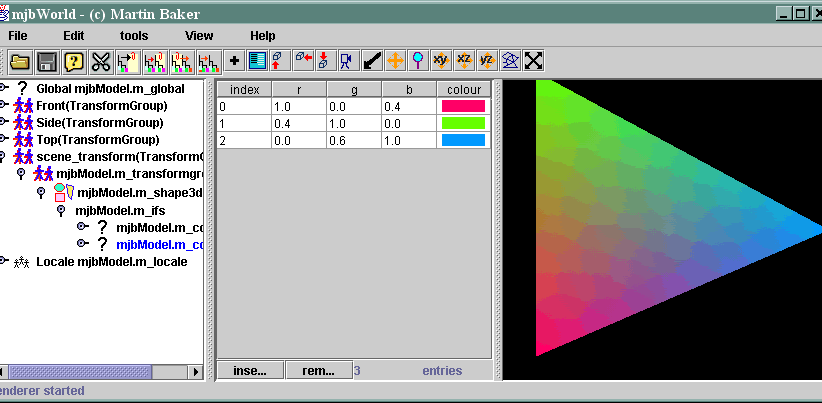## Normals

Now remove the colour entries and enter normals instead, again enter normal indexes in IFS and add a normal node.

The Normal value changes the light relected of the object depending on the direction of the normal compared to the direction of the light source.

As with colours the values in the middle of the triangle are interpolated depending on their position.to be continued:

# Building up faces into shapes

Now we can create individual faces, we can now show how to build common 3 dimentional shapes from faces.

### Box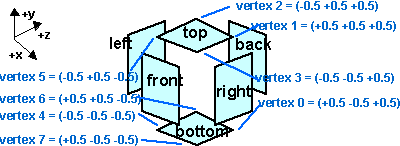• Number of faces = 6
• number of vertexes = 8
• number of edges = 12
• Vertes per face = 4
• Number of indexes = 6*4 =24

```#VRML V2.0 utf8
Transform {
scale 0.1 0.1 0.1
children [
Shape {
appearance Appearance {
material Material {diffuseColor 0.0 1.0 1.0}
}
geometry
IndexedFaceSet {
coord Coordinate {
point [
0.5 -0.5 0.5,
0.5 0.5 0.5,
-0.5 0.5 0.5,
-0.5 -0.5 0.5,
-0.5 -0.5 -0.5,
-0.5 0.5 -0.5,
0.5 0.5 -0.5,
0.5 -0.5 -0.5,  ]
}
coordIndex [
0, 1, 2, 3, -1,
4, 5, 6, 7, -1,
7, 6, 1, 0, -1,
3, 2, 5, 4, -1,
1, 6, 5, 2, -1,
3, 4, 7, 0, -1, ]
normal Normal {
vector [
0.0 0.0 1.0,
0.0 0.0 -1.0,
1.0 0.0 0.0,
-1.0 0.0 0.0,
0.0 1.0 0.0,
0.0 -1.0 0.0, ]
}
normalIndex [
0, 0, 0, 0, -1,
1, 1, 1, 1, -1,
2, 2, 2, 2, -1,
3, 3, 3, 3, -1,
4, 4, 4, 4, -1,
5, 5, 5, 5, -1,
]
texCoord TextureCoordinate {
point [
1.0 0.0,
1.0 1.0,
0.0 1.0,
0.0 0.0,
1.0 0.0,
1.0 1.0,
0.0 1.0,
0.0 0.0,]
}
texCoordIndex [
0, 1, 2, 3, -1,
4, 5, 6, 7, -1,
7, 6, 1, 0, -1,
3, 2, 5, 4, -1,
1, 6, 5, 2, -1,
3, 4, 7, 0, -1, ]
}
}
]
}
```

### Triangular Pyrimid• Number of faces = 4
• number of vertexes 4
• number of edges = 6
• Vertes per face = 3
• Number of indexes = 4*3 =12

`#VRML V2.0 utf8Transform { scale 0.1 0.1 0.1  children [   Shape   {  appearance Appearance    {   material Material {    diffuseColor 0.0 1.0 1.0    }    }   geometry    IndexedFaceSet {   coord Coordinate {     point      [     0.0 0.5 0.0,     -0.5 -0.5 -0.5,     0.5 -0.5 -0.5,     0.5 -0.5 0.5,     ]          }    coordIndex [    0, 3, 2, -1,     0, 2, 1, -1,     0, 1, 3, -1,     2, 3, 1, -1,     ]       normal Normal {     vector      [    0.20080805 0.9588286 -0.20080805,    -0.77121305 -0.602902 -0.20430288,    0.7046567 -0.083173454 -0.7046567,    0.20430288 -0.602902 0.77121305,    ]       }    normalIndex [    0, 3, 2, -1,     0, 2, 1, -1,     0, 1, 3, -1,     2, 3, 1, -1,     ]       }   }  ]  } `

here is the VRML file

here is the normals generated when normalPerFace is present and set to FALSE

this appears blank:

```   normal Normal {
vector
[
0.8944272 0.4472136 0.0,
0.0 0.4472136 -0.8944272,
-0.70710677 0.0 0.70710677,
0.0 -1.0 0.0,
]

}
normalIndex [
0, 0, 0, -1,
1, 1, 1, -1,
2, 2, 2, -1,
3, 3, 3, -1,
]

normalPerVertex FALSE
```

### Rectangular Pyrimid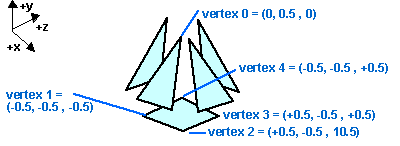• Number of faces = 5
• number of vertexes = 5
• number of edges = 8
• Vertes per face = 3 and 4
• Number of indexes = 16

Generation of indexes array out of bounds error 16 and no normals generated.

`#VRML V2.0 utf8Transform { scale 0.1 0.1 0.1  children [   Shape   {  appearance Appearance    {   material Material {    diffuseColor 0.0 1.0 1.0    }    }   geometry    IndexedFaceSet {   coord Coordinate {     point      [     0.0 0.5 0.0,     -0.5 -0.5 -0.5,     0.5 -0.5 -0.5,     0.5 -0.5 0.5,     -0.5 -0.5 0.5,     ]          }    coordIndex [    0, 3, 2, -1,     0, 2, 1, -1,     0, 1, 4, -1,     2, 4, 3, -1,     2, 3, 4, 1, -1,     ]       normalIndex [    0, 3, 2, -1,     0, 2, 1, -1,     0, 1, 4, -1,     2, 4, 3, -1,     2, 3, 4, -1,     2, ]       }   }  ]  } `

here is the VRML file

here is the normals generated when normalPerFace is present and set to FALSE

normalIndex [
0, 0, 0, -1,
3, 3, 3, -1,
2, 2, 2, -1,
0, 0, 0, -1,
2, 2, 2, -1,
1, ]

normalPerVertex FALSE

```
```

### Box minus chunk• Number of faces = 9
• number of vertexes = 12
• number of edges = 18
• Vertes per face = 4 and 6
• Number of indexes = 42
```#VRML V2.0 utf8Transform { scale 0.1 0.1 0.1  children [   Shape   {  appearance Appearance    {   material Material {    diffuseColor 0.0 1.0 1.0    }    }   geometry    IndexedFaceSet {   coord Coordinate {     point      [     0.5 -0.5 0.5,     0.5 0.5 0.5,     -0.5 0.5 0,     -0.5 0 0,     -0.5 0 -0.5,     -0.5 -0.5 -0.5,     0.5 0.5 -0.5,     0.5 -0.5 -0.5,     -0.5 -0.5 -0.5,     -0.5 0.5 -0.5,     0.5 0.5 -0.5,     0.5 -0.5 -0.5,     ]          }    coordIndex [    0, 1, 2, 3, -1,     4, 5, 6, 7, -1,     7, 6, 1, 0, -1,     3, 2, 5, 4, -1,     1, 6, 5, 2, -1,     3, 4, 7, 0, -1,     0, 1, 2, 3, -1,     4, 5, 6, 7, -1,     7, 6, 1, 0, -1,     ]          }   }  ]  }
VRML file is here
```

### Dodecahedron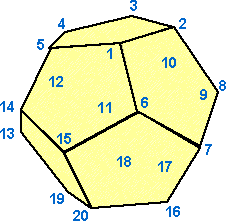• Number of faces = 12
• Edges = 30
• Vertices = 20
• Vertes per face = 5
• Number of indexes = 60
` `

### Icosahedron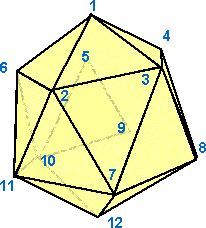• Number of faces = 20
• Edges = 30
• Vertices = 12
• Vertes per face = 3
• Number of indexes = 60